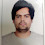Introduction

The most important question to evaluate any person in java is to ask the architecture of hashmap and how hashmap is internally working in Java.We will begin with the basic understand of hashmap.

What is HashMap?

HashMap is nothing but the implementation of Map interface in Java. HashMap is introduced in Java in Java version 1.2. HashMap is storing the data in the Key,Value Pair.The HashMap is called as HashMap because it is internally working on a technique called hashing.Another important point to note here is that the key in HashMap obejct is unique.

Internal working of hashmap

The main data structure internally used by hashmap are array and linked list(as you can see in below image).

Consider that object of hashmap is created using below code.

Map<Integer, String> hashMap = new HashMap<String,Integer,>()

Now on above object each method written on above image is called.When  put method will be called using values of the hashmap.

1. Calculate hashvalue for key1.
2. Based on hashvalue calculate the index.( hashValue & n-1) where n is size of array.
3. If at the calculated index of array there is no element then it will add the value in the first linked list node.
4. If value does exist at the calculated index using hash value, it will go to the last linked list node on particular index and will append the value.
Now consider a scenario where the get method of an object is called.In this case it will follow below things.

1. Calculate hashvalue specified in method arguments of get method.
2. Based on hashvalue calculate the index.( hashValue & n-1) where n is size of array.
3. If at the calculated index of array there is only one element then it will return that value
4. If there are multiple nodes available at the calculated index, it will first compare the hashValue and if it is the same it will compare the key using equals method.

Design of HashMap Class

You can find java file of  HashMap.java on below git link.

Inside the HashMap class, which is inside the java.util package,there is a static class named as Node containing below four fields.

1. hash => Hash value of a value calculated using hashing
2. value=>value of node.
3. key=>Key of a node
4. next=>Pointer to the next node.
Now lets see how it works, First it will do the hashing of key.What exactly hashing does is converting an object in to integer value.This value is calculated using the Hashcode method of object class.Once we have the hash value.We need to add  value of hashmap at the index which is calculated using hashing technique.
Now the problem here is that the hashing value is too long.If we add the value of hashmap at the index of hashvalue the array will become so much larger.So what hashmap does here is that, using the default size of array and bitwise operator it will calculate the index of value in array.
Once the index value is calculated ,It will create and object of node and it will add that node i linked list.IF the specified index is null the head pointer of that linked list will to this element other wise appended at the last value.

Another point to note in hashmap is about the instance variable.There are many instance variable declared in hashmap class.But the important one are table,threshold and loadfactor. table is array in which all the key,values are getting stored.threshold  is a variable whic is usefull in identification whether the array is full or not.If the array becomes full it will double the size of array.

Now if the numbers of keys added are greater than the threshold value than it will expand the map.In the put/putAll method this check has been made.

Default initial capacity of table inside hashmap class it 16.

## CONVERSATION

1.Take a look at the following example to understand its implementation –

HashMap map = new HashMap<>();
map.put("Rahul", 16);
map.put("Ravi", 15);
map.put("Sam",16);
Now if we want to calculate the index of any one entry, for example- “Sam”, we use the following formula –

Index = hashcode(Key) & (n-1)
The code for it is as follows –

Index = hashcode(Sam) & (3-1)
Implementation of HashCode in Java